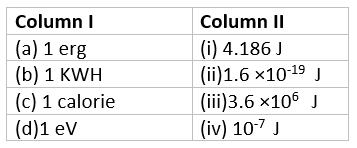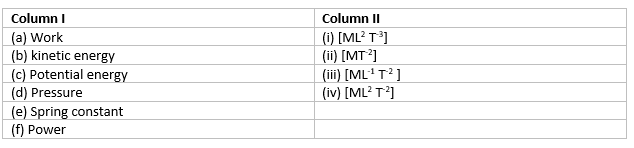# Work Energy and Power Questions and Answers

## Important Work Energy Power Questions with Answers

This page contains work, energy and power important questions which is chapter 6 of class 11 physics NCERT book. On this page you will get different types of questions to practice for

### Multiple Choice Questions on Work Energy and Power

Given below are work energy and power class 11 mcq along with their answers.

Question 1
Which of the following is equal with Newton-meter?
(a) Joule
(b) Horse Power
(c) Watt
(d) Pascal

Question 2
A particle is thrown upward with some kinetic energy. What happened to its kinetic energy at the highest point or height it reaches.
(a) Its kinetic energy is lost
(b) It�s all kinetic energy is absorbed by the air
(c) Its kinetic energy is converted to potential energy
(d) Its kinetic energy is remain same

Question 3
A body is falling freely under the action of gravity alone in vacuum.Which of the following quantities remain constant during the fall?
(a) Kinetic energy.
(b) Potential energy.
(c) Total mechanical energy.
(d) Total linear momentum.

Question 4
During inelastic collision between two bodies, which of the following quantities always remain conserved?
(a) Total kinetic energy.
(b) Total mechanical energy.
(c) Total linear momentum.
(d) Speed of each body

Question 5
What is the smallest unit of power?
(a) Watt
(b) Kilowatt
(c) Horse power
(d) Milliwatt

Question 6
A mass of 2 kg is moving along a circular path of radius 1 m. If the mass moves with 300 revolutions per minute, its kinetic energy would be
(a) $250 \pi ^2$
(b) $100\pi ^2$
(c) $5\pi ^2$
(d) 0

Question 7
If the velocity of the object is increased by 0.1%, then the kinetic energy is increased by
(a) 0.1 %
(b) 0.2%
(c) 0.4%
(d) 0.01%

Question 8
A loaf of Bread gives a boy of mass 40kg an energy of 21kJ. If the efficiency is 28% then the height can be climbed by him using this energy is
(a) 15 m
(b) 21 m
(c) 19.5m
(d) 15.5 m

Question 9
A body of mass 1 kg is rotating in a circular path of radius 1 m with constant velocity of 5 rev/sec. The work done in one complete revolution is
a. 5 J
b. 2.5 J
c.12.5 J
d. 0

Question 10
Two bodies of mass $m_1$ and $m_2$ have equal kinetic energies.What is the ratio of their linear momentum
a. $\sqrt {\frac {m_1}{m_2}}$
b. $\sqrt {\frac {m_2}{m_1}}$
c. $\sqrt {\frac {m_1 + m_2}{m_2}}$

d. $\sqrt {\frac {m_1}{m_1+ m_2}}$

Question 11
A truck and car are moving with same Kinetic energies on a straight road.Their engines are simuktanously switched off .Which one will stop at a lesser distance
a. Truck
b. Car
c. Both will stop at the same distance
d. Cannot say with the given data

### Text based Questions

Question 12
Prove that the magnitude of the ratio of difference in speed after collision to the difference in the speed before collision for a 1D elastic collision is equal to one
Question 13
One end of block of mass m resting on a smooth horizontal surface is attached to one end of an ideal spring. The other end of this spring is attached to the wall.
a. Find the expression for the Workdone by the spring force if the block is moved from an initial displacement $x_i$ to a final displacement $x_f$.
b. Show that spring force is conservative in nature
c. if the block is moved from equilibrium position through a distance $x_m$ and then released from rest. Find the maximum speed of the block
Question 14
A body of mass m moving with speed v collides elastically head-on with another body of mass m initially at rest.Show that moving body will come to stop as a result of this collision
Question 15
Draw a graph showing the variation of potential energy and kinetic energy with respect to height of a free fall under gravitational force
Question 16
State Work-energy theorem . Prove it for Variable force

### Match the Column

Question 17Question 18## Numerical Questions on Work Power and Ensrgy

Given below are numericals on work power and energy for class 11 physics chapter 6. These work energy and power class 11 numericals comes with solutions that can be checked by clicking the 'Solutions' button.

Question 19
A particle moves along the X-axix from x=0 to x=5 m under the action of the force given by
$F=7-2x+3x^2$
Find the workdone in the process

Question 20
A particle of mass m is moving in a horizontal circle of radius r, under a centripetal fortce equal to ($-k/r^2$) where k is the constant. What is the total energy of the particle?

Question 21
A car of mass 1000kg accelerates uniformly from rest to the velocity of 15 m/s in 5 sec.
Calculate
(i) Acceleration of the car
(ii) Gain in Kinetic Energy
(iii) Average power of the engine during this period

Question 22
A ball bounces to 80% of its original height.What fraction of its mechanical energy is lost in each bounce? where does this energy go?

Question 23
Find the value of p so that the vectors A=2i + pj + k and B=4i - 2j -2 k are pendicular to each other

Question 24
A body constrained to move along z-axis of a coordinate system is subjected to the constant force F given by
$\mathbf{F}=-\mathbf{i}+ 2\mathbf{j} + 3\mathbf{k}$
Where $\mathbf{i},\mathbf{j}$ and $\mathbf{k}$ are the unit vectors along x,y and z axis.
Calculate the workdone by the force in displacing the body through a distance of 5 m along the z-axis